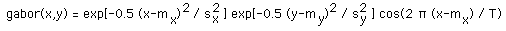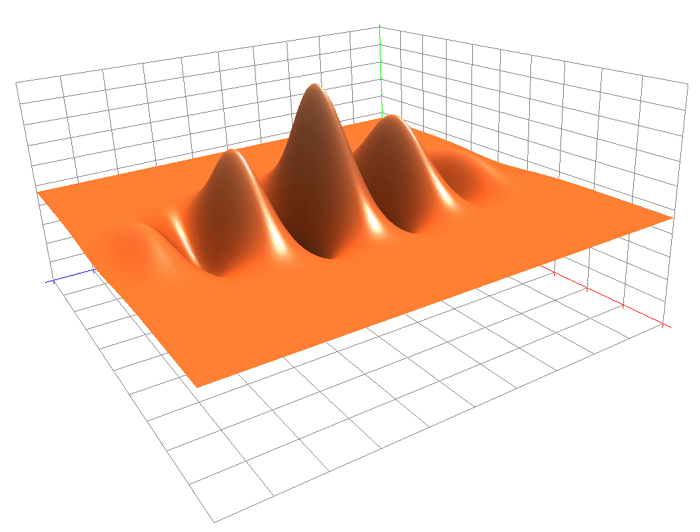## Gabor function

By JohnLast 3791 days ago Comments (7)

I used the amazing possibilities of Metatrader 5 (a real Matlab lol) to visualize the Gabor function.

I really like the result:The code to generate the Gabor equation on Metatrader 5 is:

#property version "1.00"
//--- input parameters
input double x=-3.0;
input double y=-3.0;
input int T=1;
//+------------------------------------------------------------------+
//| Tester function |
//+------------------------------------------------------------------+
double OnTester()
{
//---
double pi=3.141592653589793;
double res=MathExp (-0.5*x*x)*MathExp (-0.5*y*y)*(MathCos(2*pi*x/T));
//---
return(res);

You can see it looks the same:I let you imagine for the moment if we can use this for something usefull in Metatrader 4 or 5. As I notes there are values that are above 0 and below 0. If the output value os below 0, that will lead in practice to counter the indicator.

if the indicator is positive but the output value is negative = the result will be negative.

if the indicator is negative but the output value is positive = the result will be positive.

However under some konditions it may be usefull to actually fade the indicators. The gabor function allows exactly that, for example we want to try to cream the volatility picking for small targets with a big stop in the direction of the overall trend (this is a basic example).

• jaguar1637 3791 days ago

THis is very interesting

I am not sure the aim of this Gabor function and how to deal with. The practise should show soimething w/ Price accumulation

• jaguar1637 3791 days ago

tres sexuel ;)

• jaguar1637 3791 days ago

tres sexuel ;)

• JohnLast 3791 days ago

The gabor in 3d is even more interesting.

I am so drunk right now that it look like oignon to me lol.

• JohnLast 3791 days ago

The gabor in 3d is even more interesting. We can implement it but we can't visualize it.

I am so drunk right now that it look like oignon to me lol.

• tovim 3788 days ago

It looks interesting idea and papers describe it similar to fourrier transform.How can we use this function?

• JohnLast 3788 days ago

We can set it as a kernel for an expert. However it varies from -1 to +1, so it may not be convenient for all types of strategies.

According to what I know, for using a kernel method you need a Positive-definite kernel, and the gabor equation as far as I understand does not produce this. It is required in order to produce a dot product and to apply linear methods in the complex hyper plane (kernel trick).

However as we use a genetic optimizer we are not really bound here by this.

And even more you may be interested in some systems to counter the indications of the indicators. If you use the gaussian kernel you can't make it. But if you use the Gabor filter you can make it.

(Range bound system may be better optimized using the Gabor equation).

And it can be used to make a complex indicators. Jaguar has produced some

Any ideas are welcome.